# Binary and Hexadecimal Numbers CSE 1310 Introduction to

• Slides: 50Binary and Hexadecimal Numbers CSE 1310 – Introduction to Computers and Programming Vassilis Athitsos University of Texas at Arlington 1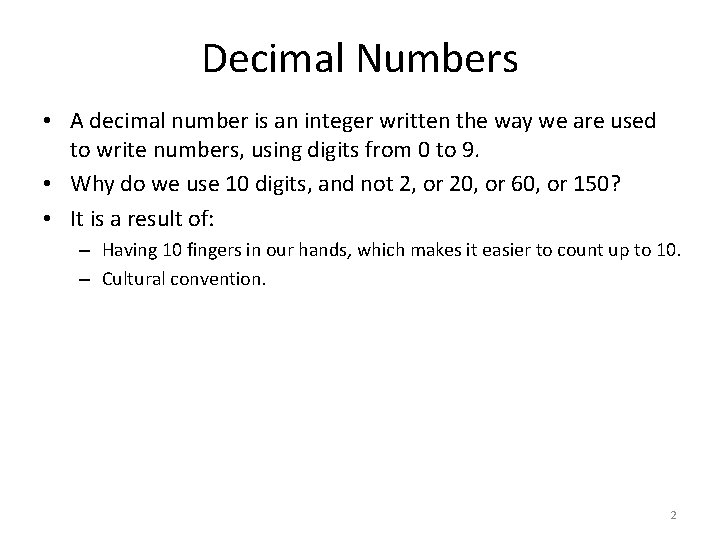Decimal Numbers • A decimal number is an integer written the way we are used to write numbers, using digits from 0 to 9. • Why do we use 10 digits, and not 2, or 20, or 60, or 150? • It is a result of: – Having 10 fingers in our hands, which makes it easier to count up to 10. – Cultural convention. 2Babylonian Numbers • Babylonians used 60 digits instead of 10. – Make sure you know them for the exam : ) 3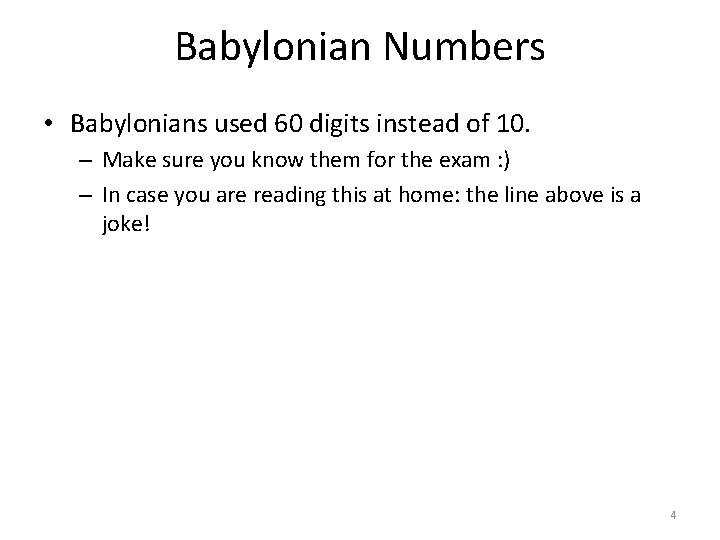Babylonian Numbers • Babylonians used 60 digits instead of 10. – Make sure you know them for the exam : ) – In case you are reading this at home: the line above is a joke! 4Babylonian Numbers • Babylonians used 60 digits instead of 10. • While we do not use that system for writing numbers today, it has survived in our modern culture. Where? • In the way we measure time. – 24 hours. – 60 minutes. – 60 seconds. • Also in the way we measure angles. – 360 degrees. – 60 minutes. – 60 seconds. 5Binary Numbers • Computers, in their electronic circuits, essentially use two digits: 1 and 0. • 1 corresponds to a certain electrical (or magnetic, or optical) signal. • 0 corresponds to another electrical (or magnetic, or optical) signal. • This means that we need to represent all numbers using those two digits. – Numbers represented using 1 and 0 are called binary numbers. • Java (like any modern programming language) allows us to use decimal numbers. • Inside the computer, before the program gets executed, all decimal numbers are translated to binary. 6Binary Numbers • Since 1310 is our introductory course, it is a good place for you to learn some basics about binary numbers. • We will do a simplified version. • We will not worry about representing: – Floating point numbers. – Negative numbers. • We will learn how to represent positive integers in binary. • We will learn how to translate from binary to decimal and the other way around. 7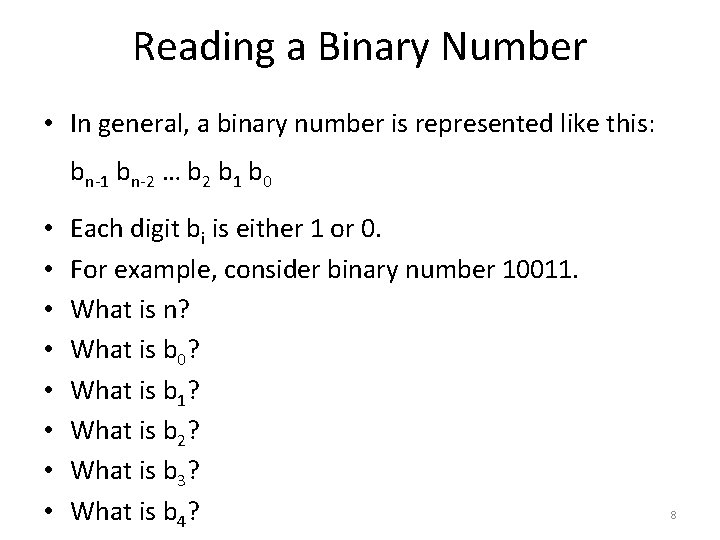Reading a Binary Number • In general, a binary number is represented like this: bn-1 bn-2 … b 2 b 1 b 0 • • Each digit bi is either 1 or 0. For example, consider binary number 10011. What is n? What is b 0? What is b 1? What is b 2? What is b 3? What is b 4? 8Reading a Binary Number • In general, a binary number is represented like this: bn-1 bn-2 … b 2 b 1 b 0 • • Each digit bi is either 1 or 0. For example, consider binary number 10011. What is n? 5 What is b 0? 1 What is b 1? 1 What is b 2? 0 What is b 3? 0 What is b 4? 1 9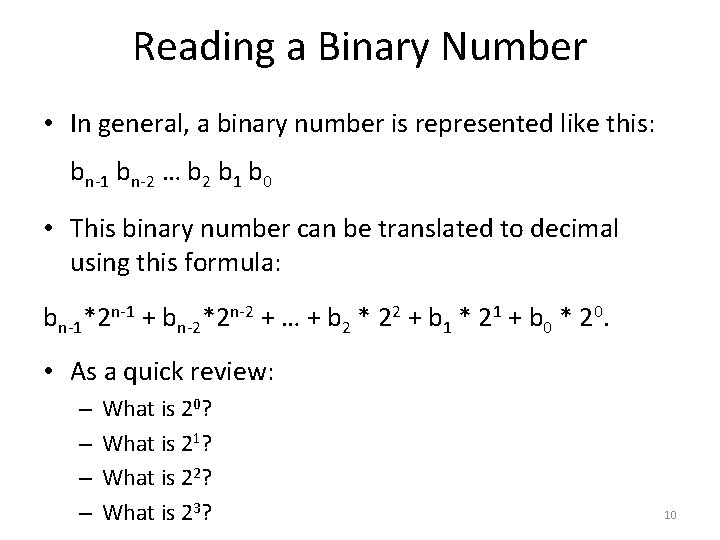Reading a Binary Number • In general, a binary number is represented like this: bn-1 bn-2 … b 2 b 1 b 0 • This binary number can be translated to decimal using this formula: bn-1*2 n-1 + bn-2*2 n-2 + … + b 2 * 22 + b 1 * 21 + b 0 * 20. • As a quick review: – – What is 20? What is 21? What is 22? What is 23? 10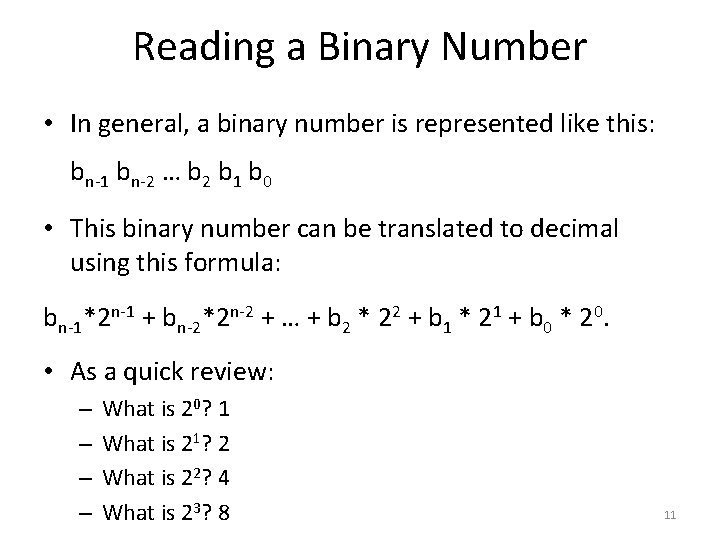Reading a Binary Number • In general, a binary number is represented like this: bn-1 bn-2 … b 2 b 1 b 0 • This binary number can be translated to decimal using this formula: bn-1*2 n-1 + bn-2*2 n-2 + … + b 2 * 22 + b 1 * 21 + b 0 * 20. • As a quick review: – – What is 20? 1 What is 21? 2 What is 22? 4 What is 23? 8 11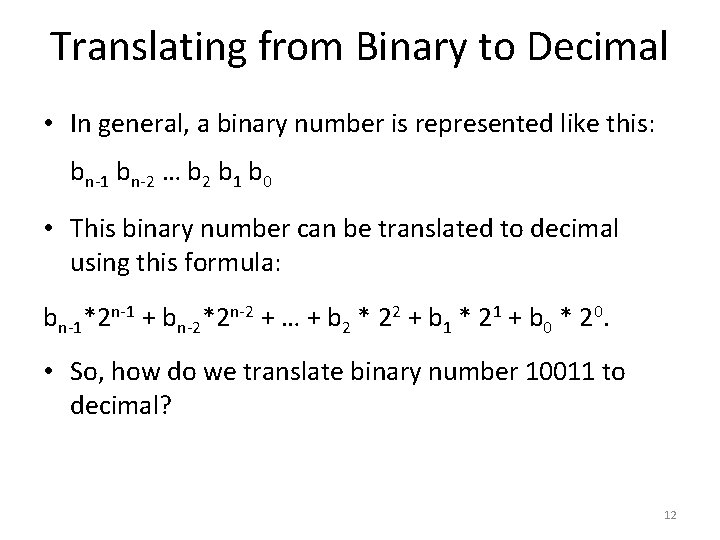Translating from Binary to Decimal • In general, a binary number is represented like this: bn-1 bn-2 … b 2 b 1 b 0 • This binary number can be translated to decimal using this formula: bn-1*2 n-1 + bn-2*2 n-2 + … + b 2 * 22 + b 1 * 21 + b 0 * 20. • So, how do we translate binary number 10011 to decimal? 12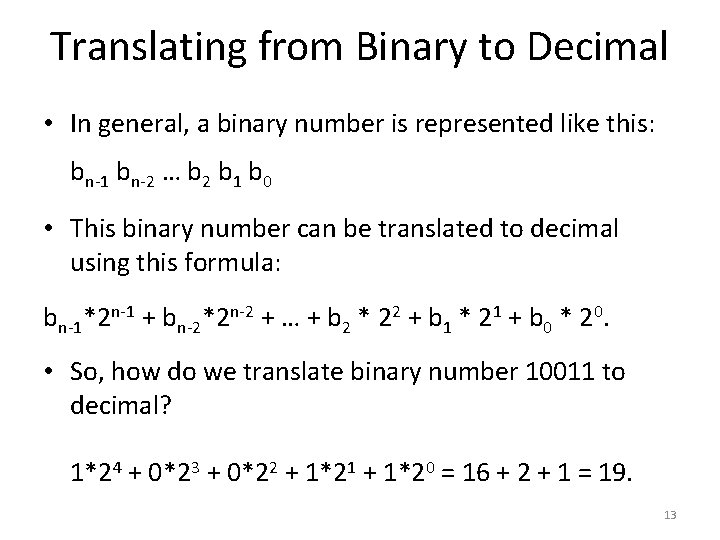Translating from Binary to Decimal • In general, a binary number is represented like this: bn-1 bn-2 … b 2 b 1 b 0 • This binary number can be translated to decimal using this formula: bn-1*2 n-1 + bn-2*2 n-2 + … + b 2 * 22 + b 1 * 21 + b 0 * 20. • So, how do we translate binary number 10011 to decimal? 1*24 + 0*23 + 0*22 + 1*21 + 1*20 = 16 + 2 + 1 = 19. 13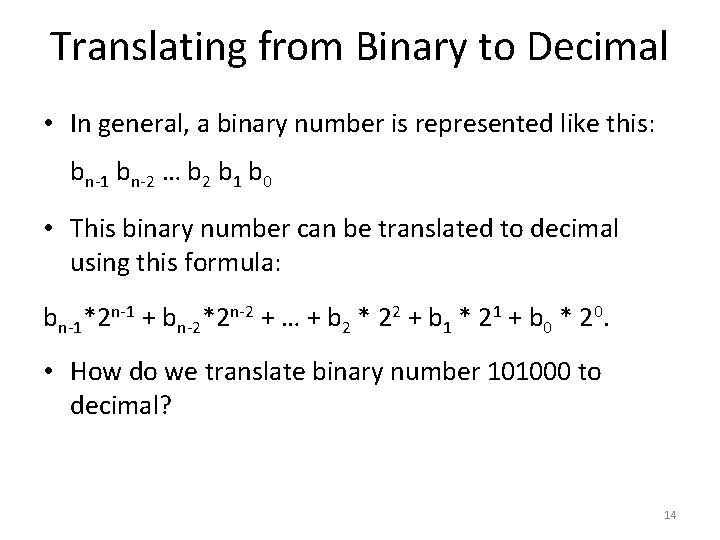Translating from Binary to Decimal • In general, a binary number is represented like this: bn-1 bn-2 … b 2 b 1 b 0 • This binary number can be translated to decimal using this formula: bn-1*2 n-1 + bn-2*2 n-2 + … + b 2 * 22 + b 1 * 21 + b 0 * 20. • How do we translate binary number 101000 to decimal? 14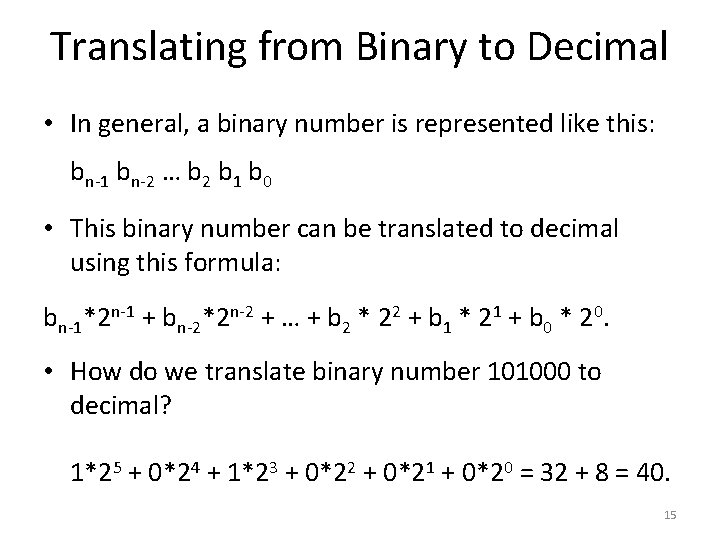Translating from Binary to Decimal • In general, a binary number is represented like this: bn-1 bn-2 … b 2 b 1 b 0 • This binary number can be translated to decimal using this formula: bn-1*2 n-1 + bn-2*2 n-2 + … + b 2 * 22 + b 1 * 21 + b 0 * 20. • How do we translate binary number 101000 to decimal? 1*25 + 0*24 + 1*23 + 0*22 + 0*21 + 0*20 = 32 + 8 = 40. 15binary. To. Decimal Function • Let's write a function binary. To. Decimal that translates a binary number into a decimal number. • What arguments should the function take? • What type should the function return? 16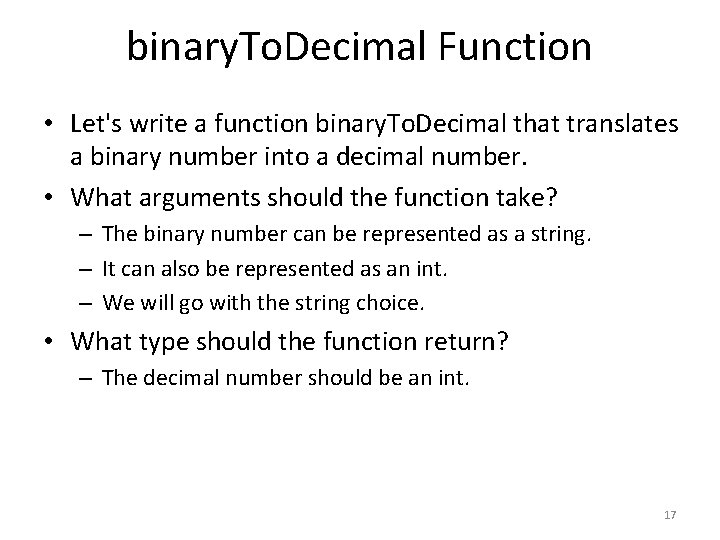binary. To. Decimal Function • Let's write a function binary. To. Decimal that translates a binary number into a decimal number. • What arguments should the function take? – The binary number can be represented as a string. – It can also be represented as an int. – We will go with the string choice. • What type should the function return? – The decimal number should be an int. 17binary. To. Decimal Function public static int binary. To. Decimal(String text) { int result = 0; for (int i = 0; i < text. length(); i++) { String c = text. substring(i, i+1); if ("01". index. Of(c) == -1) { System. out. printf("Error: invalid binary number %s, exiting. . . n", text); System. exit(0); } int digit = Integer. parse. Int(c); int power_of_2 = (int) (Math. pow(2, text. length() - i - 1)); result = result + digit * power_of_2; } return result; } 18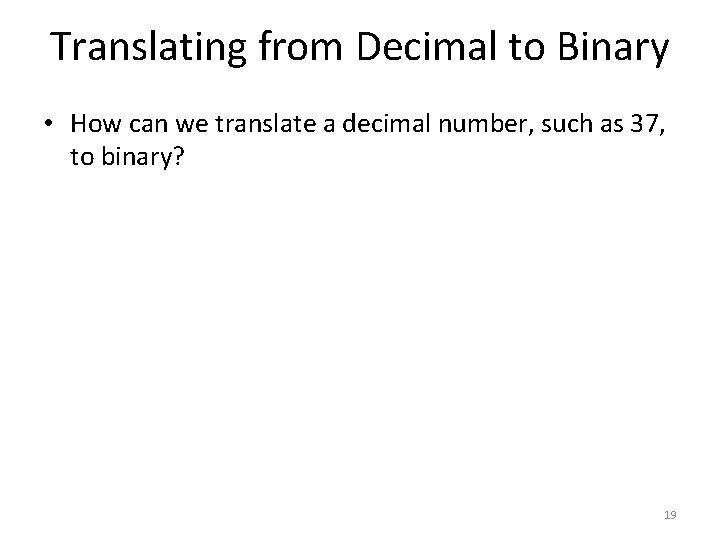Translating from Decimal to Binary • How can we translate a decimal number, such as 37, to binary? 19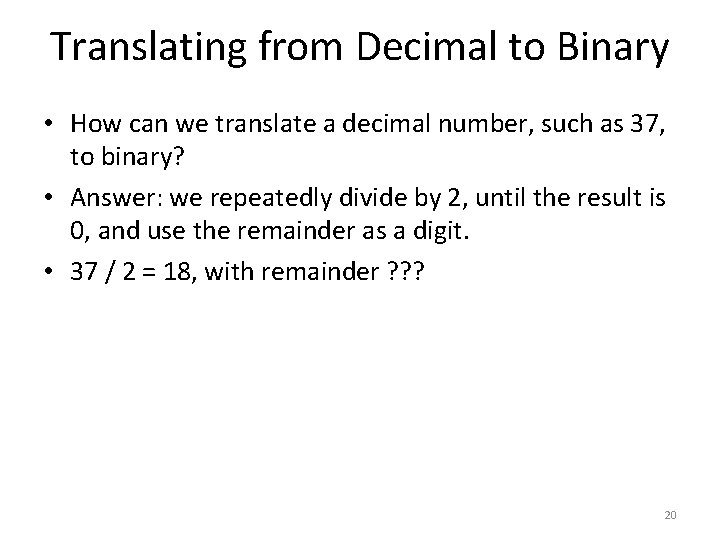Translating from Decimal to Binary • How can we translate a decimal number, such as 37, to binary? • Answer: we repeatedly divide by 2, until the result is 0, and use the remainder as a digit. • 37 / 2 = 18, with remainder ? ? ? 20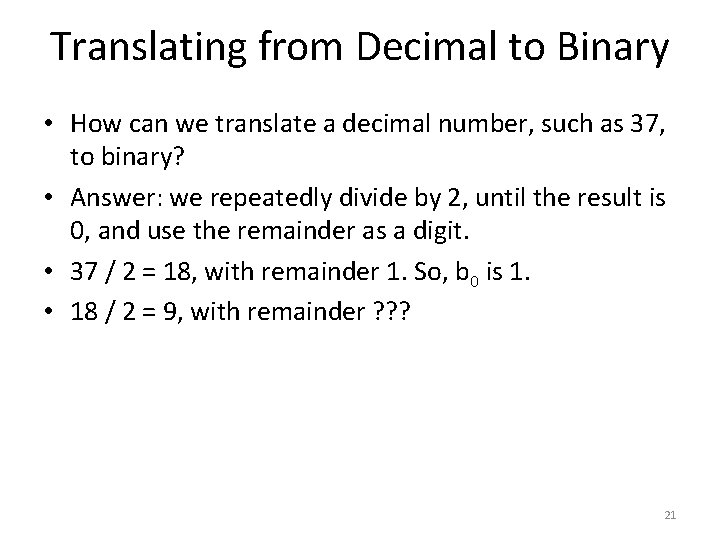Translating from Decimal to Binary • How can we translate a decimal number, such as 37, to binary? • Answer: we repeatedly divide by 2, until the result is 0, and use the remainder as a digit. • 37 / 2 = 18, with remainder 1. So, b 0 is 1. • 18 / 2 = 9, with remainder ? ? ? 21Translating from Decimal to Binary • How can we translate a decimal number, such as 37, to binary? • Answer: we repeatedly divide by 2, until the result is 0, and use the remainder as a digit. • 37 / 2 = 18, with remainder 1. So, b 0 is 1. • 18 / 2 = 9, with remainder 0. So, b 1 is 0. • 9 / 2 = 4, with remainder ? ? ? 22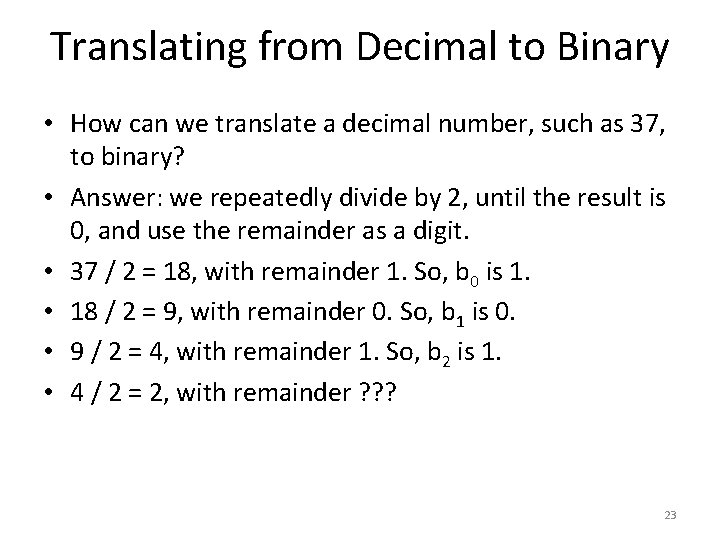Translating from Decimal to Binary • How can we translate a decimal number, such as 37, to binary? • Answer: we repeatedly divide by 2, until the result is 0, and use the remainder as a digit. • 37 / 2 = 18, with remainder 1. So, b 0 is 1. • 18 / 2 = 9, with remainder 0. So, b 1 is 0. • 9 / 2 = 4, with remainder 1. So, b 2 is 1. • 4 / 2 = 2, with remainder ? ? ? 23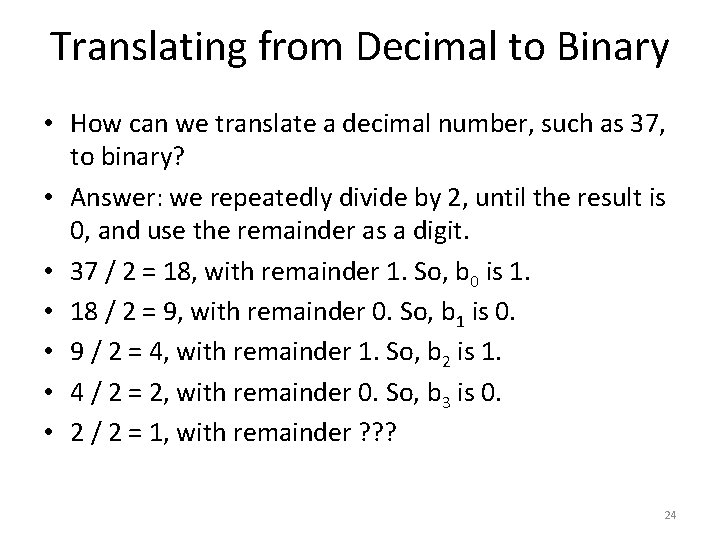Translating from Decimal to Binary • How can we translate a decimal number, such as 37, to binary? • Answer: we repeatedly divide by 2, until the result is 0, and use the remainder as a digit. • 37 / 2 = 18, with remainder 1. So, b 0 is 1. • 18 / 2 = 9, with remainder 0. So, b 1 is 0. • 9 / 2 = 4, with remainder 1. So, b 2 is 1. • 4 / 2 = 2, with remainder 0. So, b 3 is 0. • 2 / 2 = 1, with remainder ? ? ? 24Translating from Decimal to Binary • How can we translate a decimal number, such as 37, to binary? • Answer: we repeatedly divide by 2, until the result is 0, and use the remainder as a digit. • 37 / 2 = 18, with remainder 1. So, b 0 is 1. • 18 / 2 = 9, with remainder 0. So, b 1 is 0. • 9 / 2 = 4, with remainder 1. So, b 2 is 1. • 4 / 2 = 2, with remainder 0. So, b 3 is 0. • 2 / 2 = 1, with remainder 0. So, b 4 is 0. • 1 / 2 = 0, with remainder ? ? ? 25Translating from Decimal to Binary • How can we translate a decimal number, such as 37, to binary? • Answer: we repeatedly divide by 2, until the result is 0, and use the remainder as a digit. • 37 / 2 = 18, with remainder 1. So, b 0 is 1. • 18 / 2 = 9, with remainder 0. So, b 1 is 0. • 9 / 2 = 4, with remainder 1. So, b 2 is 1. • 4 / 2 = 2, with remainder 0. So, b 3 is 0. • 2 / 2 = 1, with remainder 0. So, b 4 is 0. • 1 / 2 = 0, with remainder 1. So, b 5 is 1. 26 • So, 37 in binary is ? ? ?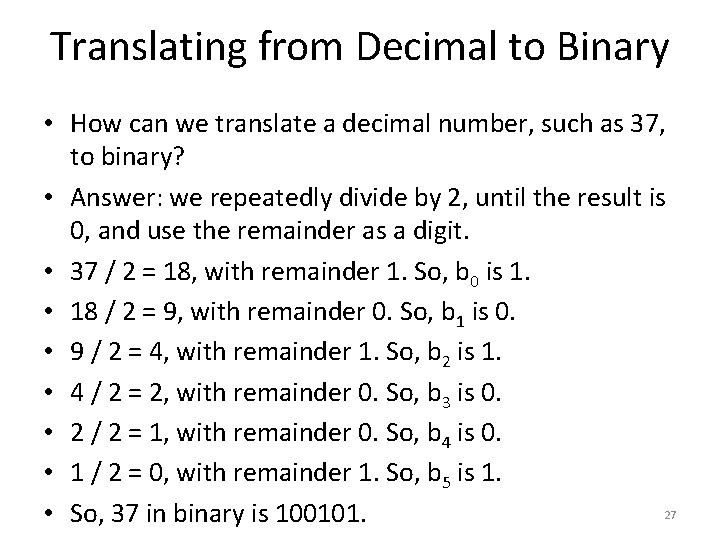Translating from Decimal to Binary • How can we translate a decimal number, such as 37, to binary? • Answer: we repeatedly divide by 2, until the result is 0, and use the remainder as a digit. • 37 / 2 = 18, with remainder 1. So, b 0 is 1. • 18 / 2 = 9, with remainder 0. So, b 1 is 0. • 9 / 2 = 4, with remainder 1. So, b 2 is 1. • 4 / 2 = 2, with remainder 0. So, b 3 is 0. • 2 / 2 = 1, with remainder 0. So, b 4 is 0. • 1 / 2 = 0, with remainder 1. So, b 5 is 1. 27 • So, 37 in binary is 100101.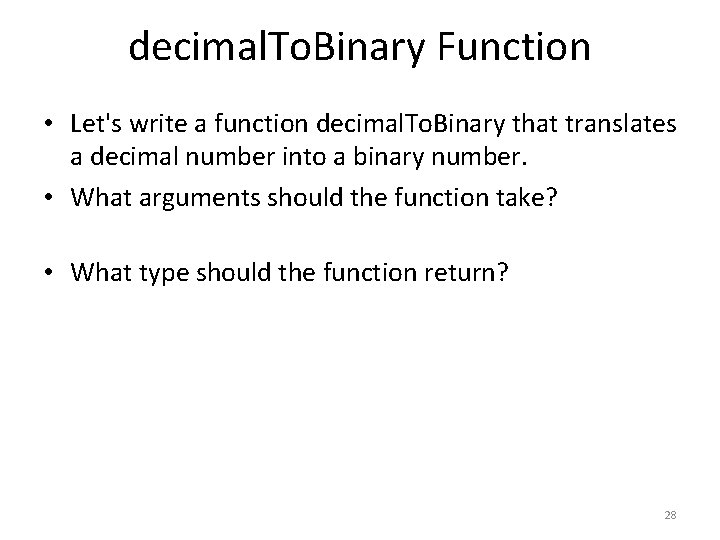decimal. To. Binary Function • Let's write a function decimal. To. Binary that translates a decimal number into a binary number. • What arguments should the function take? • What type should the function return? 28decimal. To. Binary Function • Let's write a function decimal. To. Binary that translates a decimal number into a binary number. • What arguments should the function take? – The decimal number should be an int. • What type should the function return? – Again, the most simple choice is to represent the binary number as a string. 29decimal. To. Binary Function public static String decimal. To. Binary(int number) { String result = ""; while(true) { int remainder = number % 2; String digit = Integer. to. String(remainder); result = digit + result; number = number / 2; if (number == 0) { break; } } return result; } 30Hexadecimal Numbers • Another very popular way to represent numbers among programmers is hexadecimal numbers. • Hexadecimal numbers use 16 digits: 0123456789 abcdef • • • a stands for 10. b stands for 11. c stands for 12. d stands for 13. e stands for 14. f stands for 15. 31Reading a Hexadecimal Number • A hexadecimal number is represented like this: hn-1 hn-2 … h 2 h 1 h 0 • Each digit hi is one of the sixteen digits: – 0123456789 abcdef. • • • For example, consider hexadecimal number 5 b 7. What is n? What is h 0? What is h 1? What is h 2? 32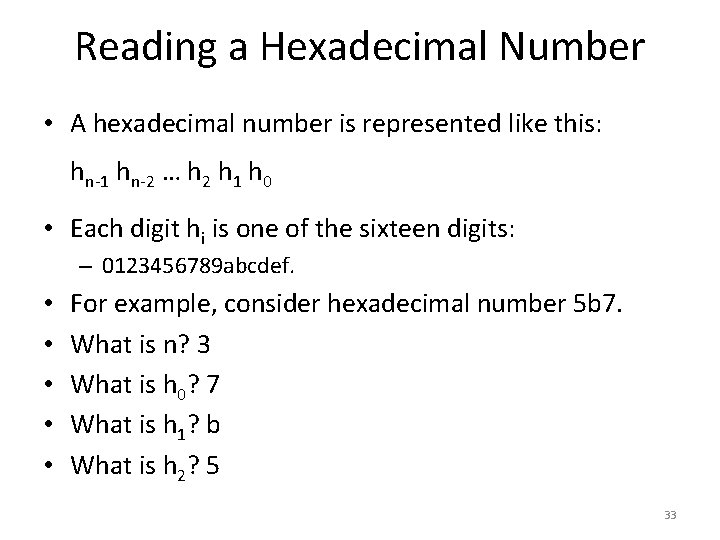Reading a Hexadecimal Number • A hexadecimal number is represented like this: hn-1 hn-2 … h 2 h 1 h 0 • Each digit hi is one of the sixteen digits: – 0123456789 abcdef. • • • For example, consider hexadecimal number 5 b 7. What is n? 3 What is h 0? 7 What is h 1? b What is h 2? 5 33Reading a Hexadecimal Number • A hexadecimal number is represented like this: hn-1 hn-2 … h 2 h 1 h 0 • This hexadecimal number can be translated to decimal using this formula: bn-1*16 n-1 + bn-2*16 n-2 + … + b 2 * 162 + b 1 * 161 + b 0*160. • Note: if bi is one of abcdef, use the corresponding number (10, 11, 12, 13, 14, 15) when you plug it into the formula. • This looks like the formula for binary numbers, except 34 that here we use powers of 16 instead of powers of 2.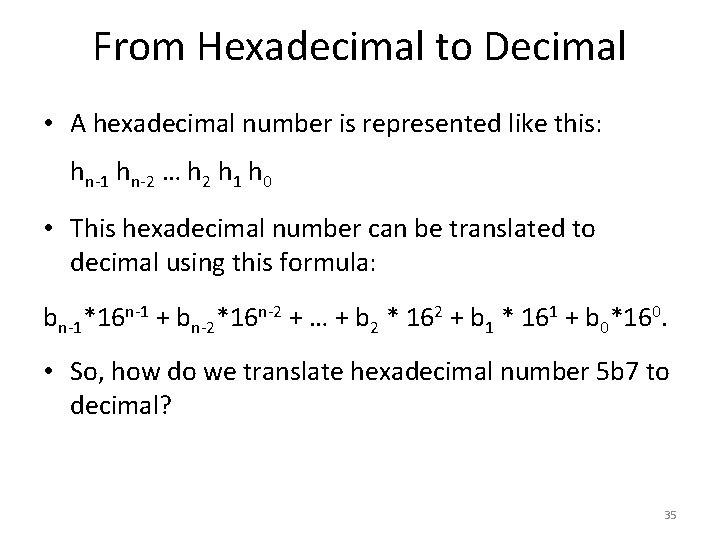From Hexadecimal to Decimal • A hexadecimal number is represented like this: hn-1 hn-2 … h 2 h 1 h 0 • This hexadecimal number can be translated to decimal using this formula: bn-1*16 n-1 + bn-2*16 n-2 + … + b 2 * 162 + b 1 * 161 + b 0*160. • So, how do we translate hexadecimal number 5 b 7 to decimal? 35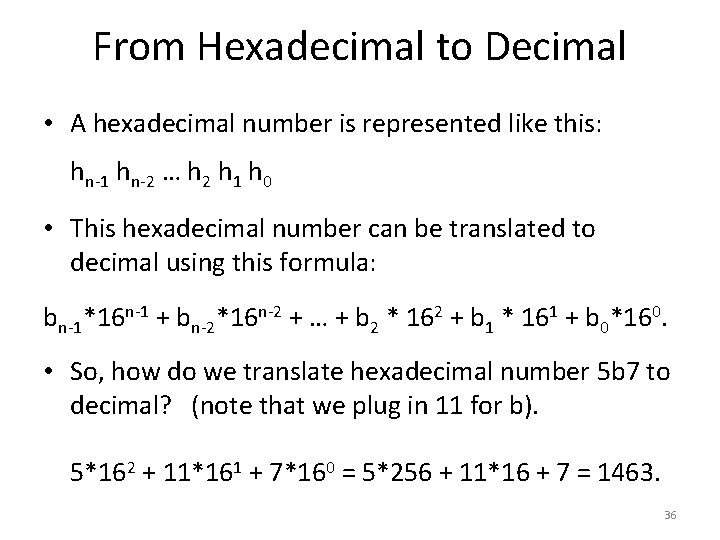From Hexadecimal to Decimal • A hexadecimal number is represented like this: hn-1 hn-2 … h 2 h 1 h 0 • This hexadecimal number can be translated to decimal using this formula: bn-1*16 n-1 + bn-2*16 n-2 + … + b 2 * 162 + b 1 * 161 + b 0*160. • So, how do we translate hexadecimal number 5 b 7 to decimal? (note that we plug in 11 for b). 5*162 + 11*161 + 7*160 = 5*256 + 11*16 + 7 = 1463. 36From Hexadecimal to Decimal • A hexadecimal number is represented like this: hn-1 hn-2 … h 2 h 1 h 0 • This hexadecimal number can be translated to decimal using this formula: bn-1*16 n-1 + bn-2*16 n-2 + … + b 2 * 162 + b 1 * 161 + b 0*160. • So, how do we translate hexadecimal number bad to decimal? 37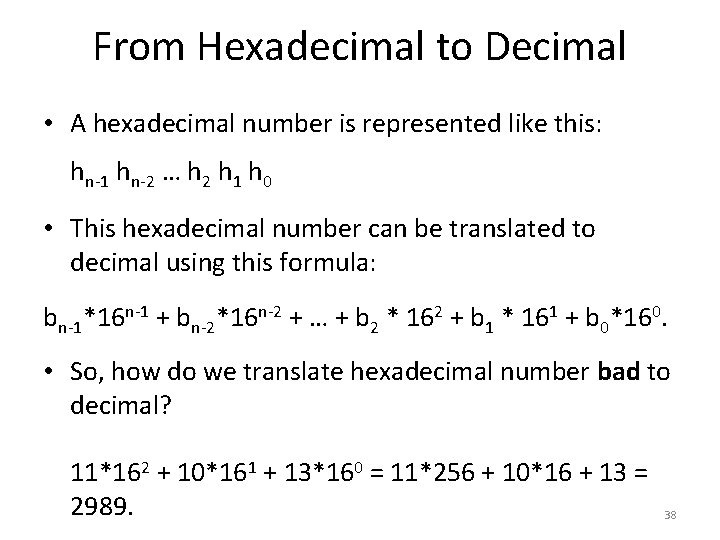From Hexadecimal to Decimal • A hexadecimal number is represented like this: hn-1 hn-2 … h 2 h 1 h 0 • This hexadecimal number can be translated to decimal using this formula: bn-1*16 n-1 + bn-2*16 n-2 + … + b 2 * 162 + b 1 * 161 + b 0*160. • So, how do we translate hexadecimal number bad to decimal? 11*162 + 10*161 + 13*160 = 11*256 + 10*16 + 13 = 2989. 38hex. To. Decimal Function • Let's write a function hex. To. Decimal that translates a hexadecimal number into a decimal number. • What arguments should the function take? • What type should the function return? 39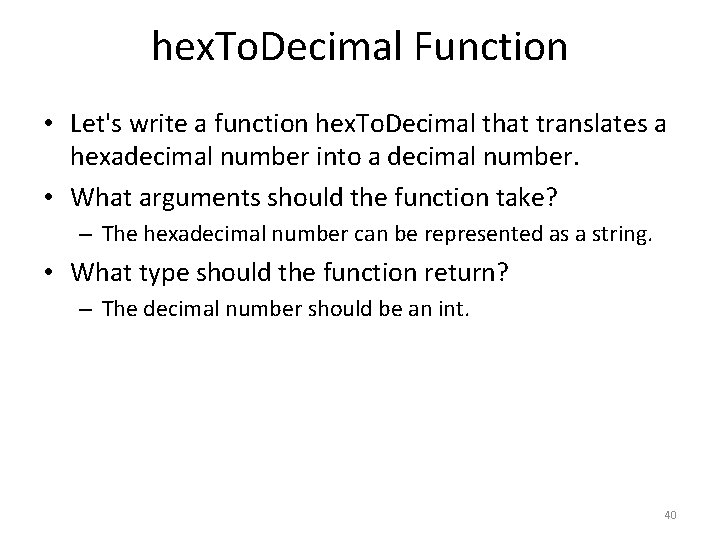hex. To. Decimal Function • Let's write a function hex. To. Decimal that translates a hexadecimal number into a decimal number. • What arguments should the function take? – The hexadecimal number can be represented as a string. • What type should the function return? – The decimal number should be an int. 40hex. To. Decimal Function public static int hex. To. Decimal(String text) { int result = 0; String digits = "0123456789 abcdef"; for (int i = 0; i < text. length(); i++) { String c = text. substring(i, i+1); int digit = digits. index. Of(c); if (digit == -1) { System. out. printf("Error: invalid hexadecimal number %s, exiting. . . n", text); System. exit(0); } int power = (int) (Math. pow(16, text. length() - i - 1)); result = result + digit * power; } return result; } 41From Decimal to Hexadecimal • How can we translate a decimal number, such as 540, to hexadecimal? 42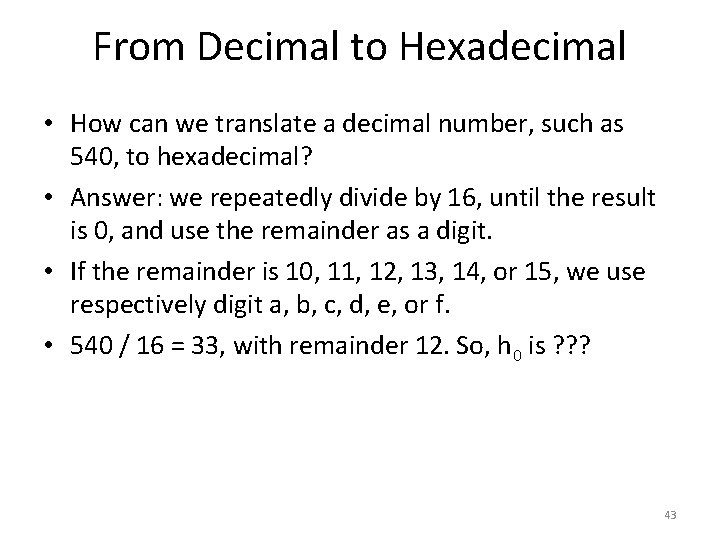From Decimal to Hexadecimal • How can we translate a decimal number, such as 540, to hexadecimal? • Answer: we repeatedly divide by 16, until the result is 0, and use the remainder as a digit. • If the remainder is 10, 11, 12, 13, 14, or 15, we use respectively digit a, b, c, d, e, or f. • 540 / 16 = 33, with remainder 12. So, h 0 is ? ? ? 43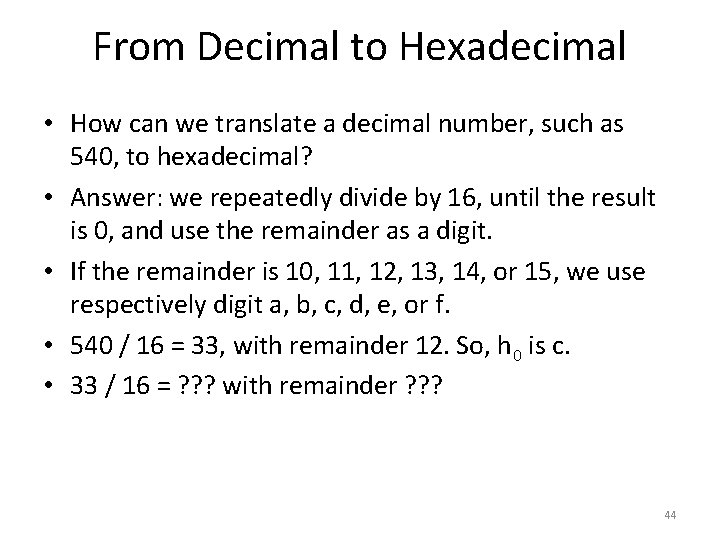From Decimal to Hexadecimal • How can we translate a decimal number, such as 540, to hexadecimal? • Answer: we repeatedly divide by 16, until the result is 0, and use the remainder as a digit. • If the remainder is 10, 11, 12, 13, 14, or 15, we use respectively digit a, b, c, d, e, or f. • 540 / 16 = 33, with remainder 12. So, h 0 is c. • 33 / 16 = ? ? ? with remainder ? ? ? 44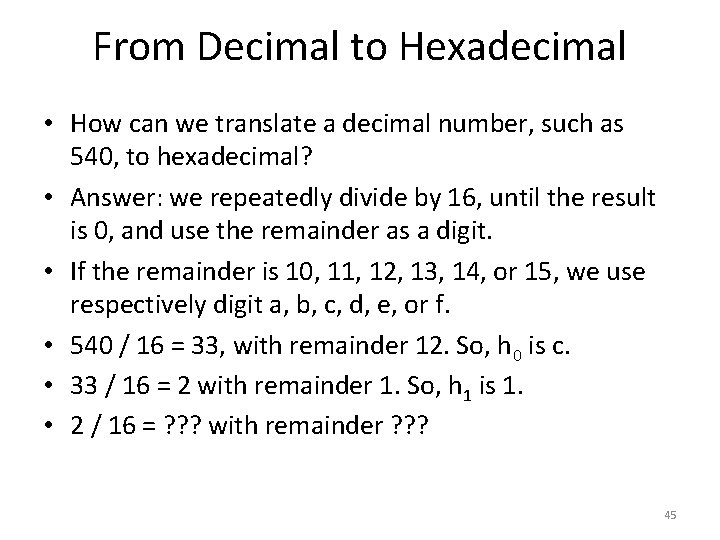From Decimal to Hexadecimal • How can we translate a decimal number, such as 540, to hexadecimal? • Answer: we repeatedly divide by 16, until the result is 0, and use the remainder as a digit. • If the remainder is 10, 11, 12, 13, 14, or 15, we use respectively digit a, b, c, d, e, or f. • 540 / 16 = 33, with remainder 12. So, h 0 is c. • 33 / 16 = 2 with remainder 1. So, h 1 is 1. • 2 / 16 = ? ? ? with remainder ? ? ? 45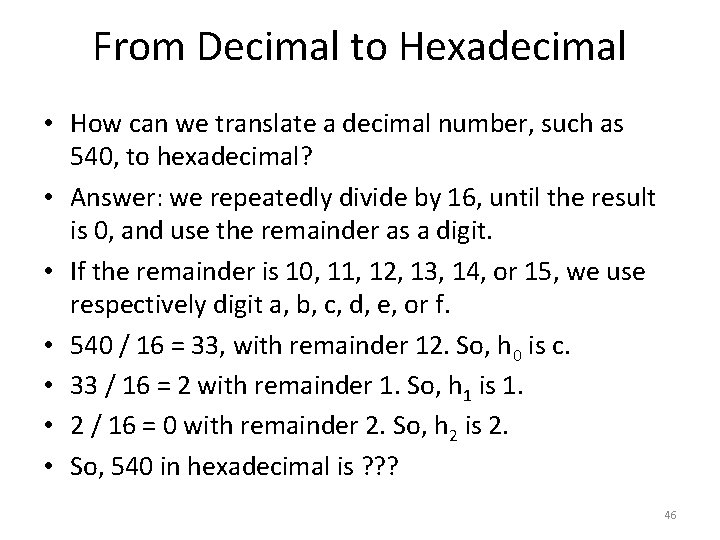From Decimal to Hexadecimal • How can we translate a decimal number, such as 540, to hexadecimal? • Answer: we repeatedly divide by 16, until the result is 0, and use the remainder as a digit. • If the remainder is 10, 11, 12, 13, 14, or 15, we use respectively digit a, b, c, d, e, or f. • 540 / 16 = 33, with remainder 12. So, h 0 is c. • 33 / 16 = 2 with remainder 1. So, h 1 is 1. • 2 / 16 = 0 with remainder 2. So, h 2 is 2. • So, 540 in hexadecimal is ? ? ? 46From Decimal to Hexadecimal • How can we translate a decimal number, such as 540, to hexadecimal? • Answer: we repeatedly divide by 16, until the result is 0, and use the remainder as a digit. • If the remainder is 10, 11, 12, 13, 14, or 15, we use respectively digit a, b, c, d, e, or f. • 540 / 16 = 33, with remainder 12. So, h 0 is c. • 33 / 16 = 2 with remainder 1. So, h 1 is 1. • 2 / 16 = 0 with remainder 2. So, h 2 is 2. • So, 540 in hexadecimal is 21 c. 47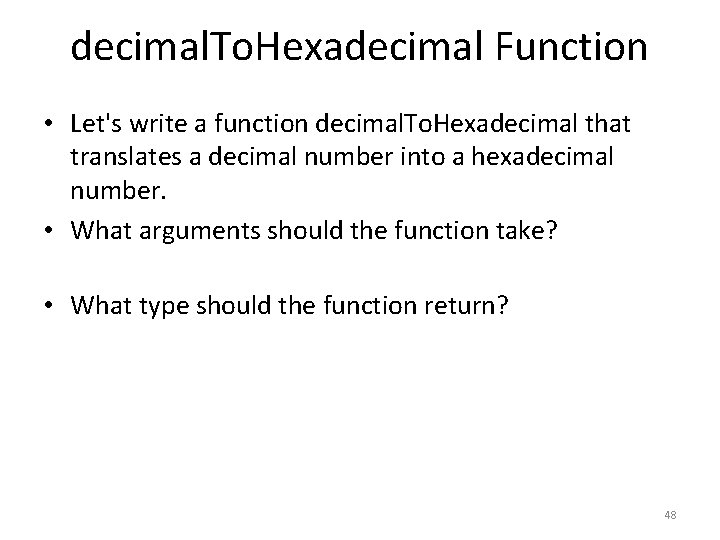decimal. To. Hexadecimal Function • Let's write a function decimal. To. Hexadecimal that translates a decimal number into a hexadecimal number. • What arguments should the function take? • What type should the function return? 48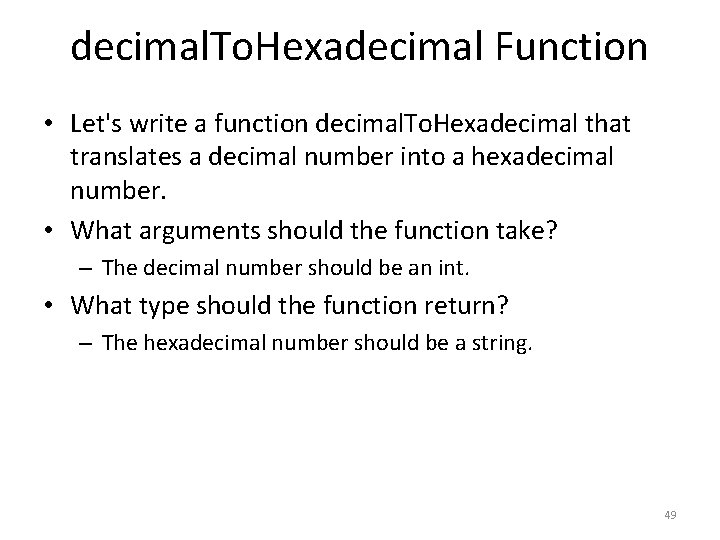decimal. To. Hexadecimal Function • Let's write a function decimal. To. Hexadecimal that translates a decimal number into a hexadecimal number. • What arguments should the function take? – The decimal number should be an int. • What type should the function return? – The hexadecimal number should be a string. 49decimal. To. Hexadecimal Function public static String decimal. To. Hexadecimal(int number) { String result = ""; String digits = "0123456789 abcdef"; while(true) { int remainder = number % 16; String digit = digits. substring(remainder, remainder+1); result = digit + result; number = number / 16; if (number == 0) { break; } } return result; } 50